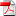# Deep backward multistep schemes for nonlinear PDEs and approximation error analysis - M. Germain, H. Pham & X. Warin

1
Juil

We develop multistep machine learning schemes for solving nonlinear partial differential equations (PDEs) in high dimension. The method is based on probabilistic representation of PDEs by backward stochastic differential equations (BSDEs) and its iterated time discretization. In the case of semilinear PDEs, our algorithm estimates simultaneously by backward induction the solution and its gradient by neural networks through sequential minimizations of suitable quadratic loss functions that are performed by stochastic gradient descent. The approach is extended to the more challenging case of fully nonlinear PDEs, and we propose different approximations of the Hessian of the solution to the PDE, i.e., the T-component of the BSDE, by combining Malliavin weights and neural networks. Extensive numerical tests are carried out with various examples of semilinear PDEs including viscous Burgers equation and examples of fully nonlinear PDEs like Hamilton-Jacobi-Bellman equations arising in portfolio selection problems with stochastic volatilities, or Monge-Ampère equations in dimension up to 15. The performance and accuracy of our numerical results are compared with some other recent machine learning algorithms in the literature, see [HJE17], [HPW20], [BEJ19], [Bec+19] and [PWG19]. Furthermore, we provide a rigorous approximation error analysis of the deep backward multistep scheme as well as the deep splitting method for semilinear PDEs, which yields convergence rate in terms of the number of neurons for shallow neural networks.

•Multistep